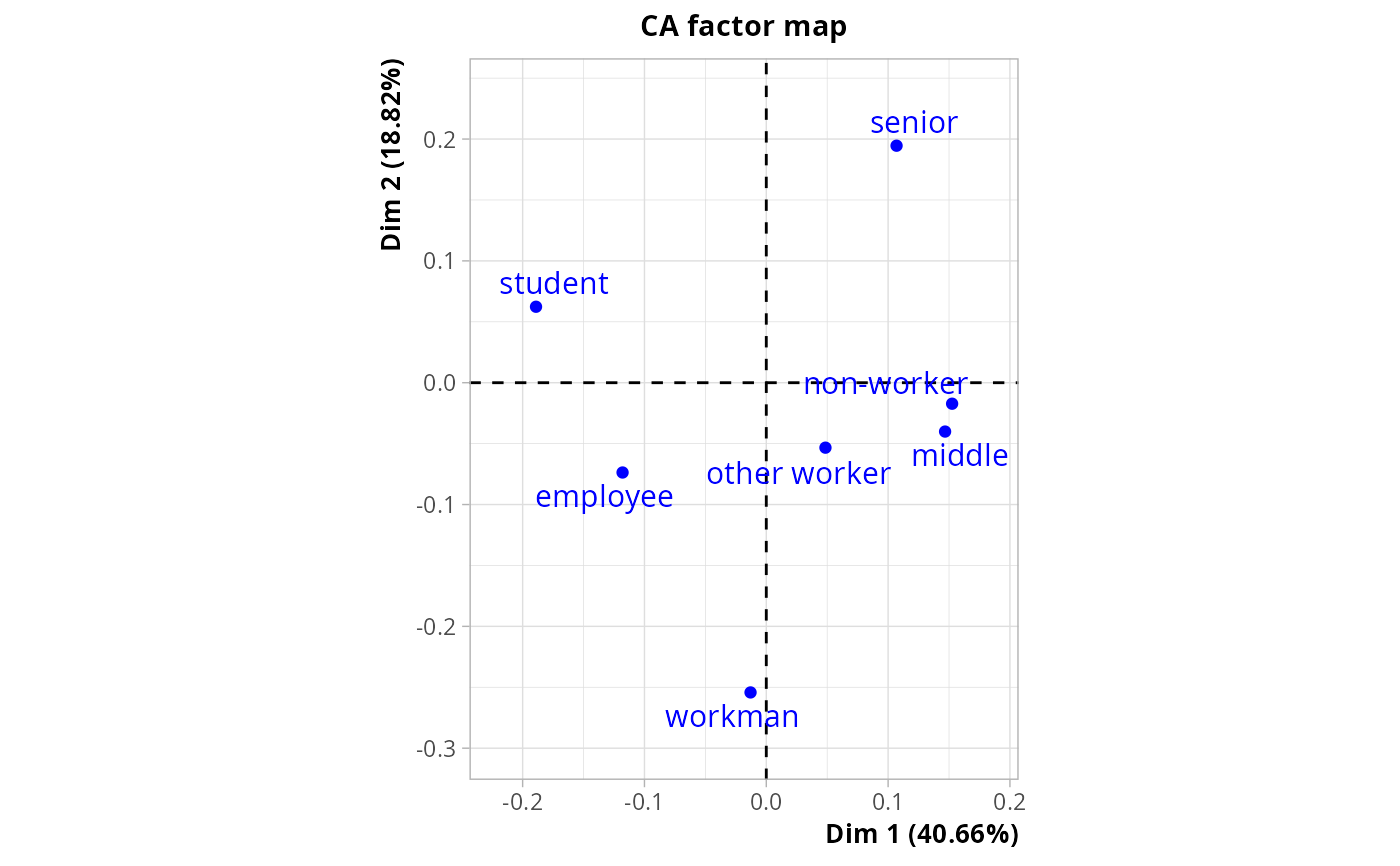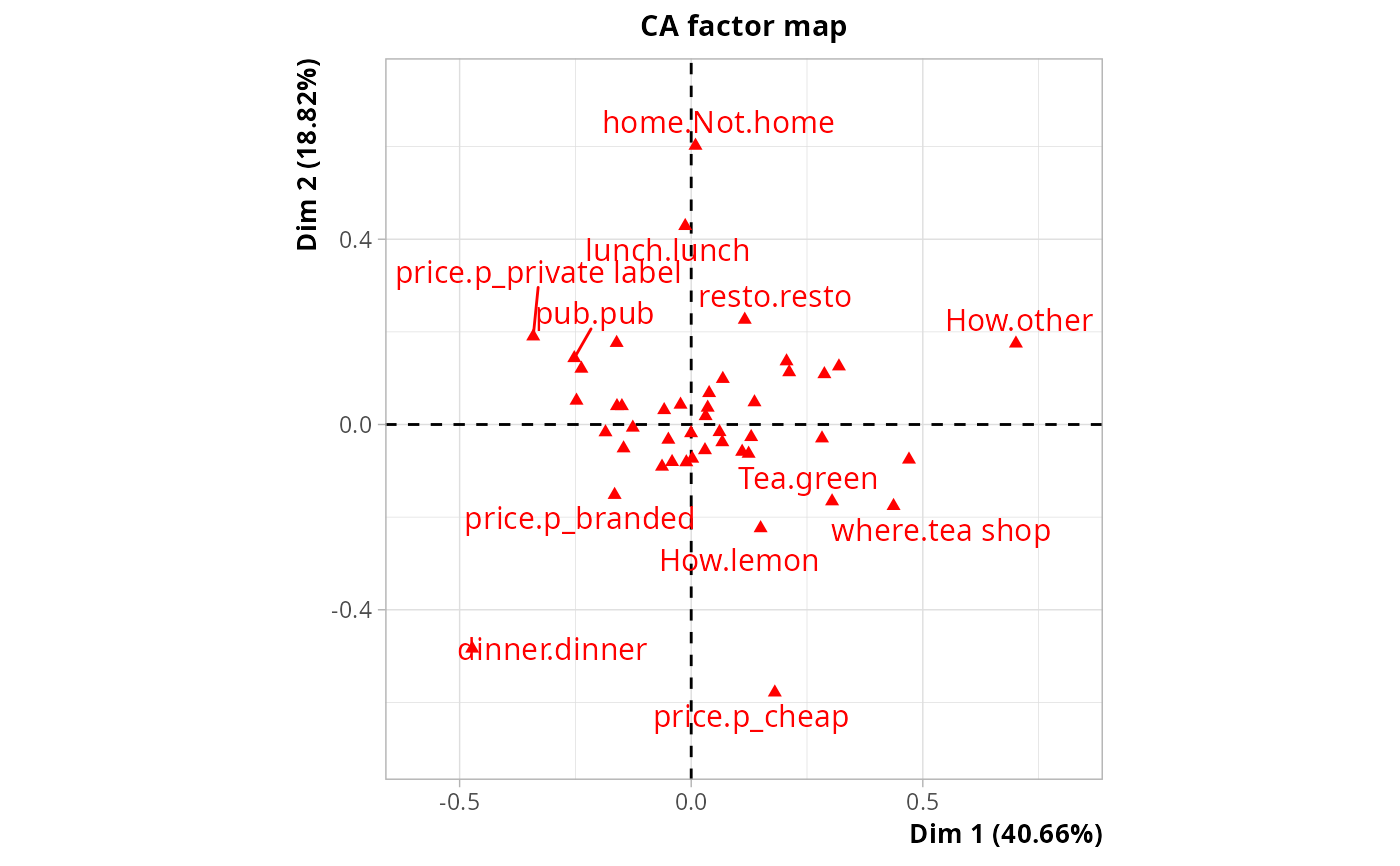Between-class MCA, also called Barycentric Discriminant Analysis

bcMCA(data, class, excl = NULL, row.w = NULL, ncp = 5)

## Arguments

data

data frame with only categorical variables, i.e. factors

class

factor specifying the class

excl

numeric vector indicating the indexes of the "junk" categories (default is NULL). See getindexcat or use ijunk interactive function to identify these indexes. It may also be a character vector of junk categories, specified in the form "namevariable.namecategory" (for instance "gender.male").

row.w

numeric vector of row weights. If NULL (default), a vector of 1 for uniform row weights is used.

ncp

number of dimensions kept in the results (by default 5)

## Details

Between-class MCA is sometimes also called Barycentric Discriminant Analysis or Discriminant Correspondence Analysis. It consists in three steps : 1. Transformation of data into an indicator matrix (i.e. disjunctive table) 2. Computation of the barycenter of the transformed data for each category of class 3. Correspondence Analysis of the set of barycenters Between-class MCA can also be viewed as a special case of MCA with instrumental variables, with only one categorical instrumental variable.

## Value

An object of class CA from FactoMineR package, with the indicator matrix of data as supplementary rows, and an additional item :

ratio

the between-class inertia percentage

## Author

Nicolas Robette

DAQ, MCAiv
library(FactoMineR)
res <- bcMCA(tea[,1:18], tea$SPC) # categories of class plot(res, invisible = c("col", "row.sup"))# Variables in tea data plot(res, invisible = c("row", "row.sup")) #> Warning: ggrepel: 26 unlabeled data points (too many overlaps). Consider increasing max.overlaps# between-class inertia percentage res$ratio